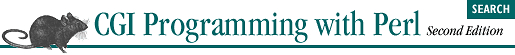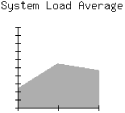home | O'Reilly's CD bookshelfs | FreeBSD | Linux | Cisco | Cisco Exam## 13.3. Generating PNGs with GD

### 13.3.1. Installation

The gd library is available at http://www.boutell.com/. This site also has instructions for building gd plus references to other optional packages that gd uses if available, such as the FreeType engine, which enables gd (and thus GD) to support TrueType fonts. Note that gd requires the latest versions of libpng and zlib; you can find links to these libraries at http://www.boutell.com/ too.

### 13.3.2. Using GD

In this section, we'll develop an application that uses the uptime Unix system command to plot the system load average (see Figure 13-1). As we will see in the next section, there are modules to help us generate graphs more easily, but let's first see gd 's graphics primitives in action.#### Figure 13-1. Sample graph generated by loads.cgi

The application itself is rather straightforward. First, we invoke the uptime command, which returns three values, representing the load averages for the previous 5, 10 and 15 minutes, respectively -- though this may differ among the various Unix implementations. Here is the output of an uptime command:

`2:26pm  up  11:07,  12 users,  load average: 4.63, 5.29, 2.56`

Example 13-2 shows the code.

```#!/usr/bin/perl -wT

use strict;

use CGI;
use GD;

BEGIN {
\$ENV{PATH} = '/bin:/usr/bin:/usr/ucb:/usr/local/bin';
delete @ENV{ qw( IFS CDPATH ENV BASH_ENV ) };
}

use constant IMAGE_SIZE     => 170;     # height and width
use constant GRAPH_SIZE     => 100;     # height and width
use constant TICK_LENGTH    => 3;

use constant ORIGIN_X_COORD => 30;
use constant ORIGIN_Y_COORD => 150;

use constant TITLE_TEXT     => "System Load Average";
use constant TITLE_X_COORD  => 10;
use constant TITLE_Y_COORD  => 15;

use constant AREA_COLOR     => ( 255, 0, 0 );
use constant AXIS_COLOR     => ( 0, 0, 0 );
use constant TEXT_COLOR     => ( 0, 0, 0 );
use constant BG_COLOR       => ( 255, 255, 255 );

my \$q     = new CGI;

print \$q->header( -type => "image/png", -expires => "-1d" );

binmode STDOUT;

# Returns a list of the average loads from the system's uptime command
my \$uptime = `uptime` or die "Error running uptime: \$!";
my( \$up_string ) = \$uptime =~ /average: (.+)\$/;
split /,\s*/, \$up_string;
@loads or die "Cannot parse response from uptime: \$up_string";
}

# Takes a one-dimensional list of data and returns an area graph as PNG
sub area_graph {
my \$data = shift;

my \$image = new GD::Image( IMAGE_SIZE, IMAGE_SIZE );
my \$background = \$image->colorAllocate( BG_COLOR );
my \$area_color = \$image->colorAllocate( AREA_COLOR );
my \$axis_color = \$image->colorAllocate( AXIS_COLOR );
my \$text_color = \$image->colorAllocate( TEXT_COLOR );

\$image->string( gdLargeFont, TITLE_X_COORD, TITLE_Y_COORD,
TITLE_TEXT, \$text_color );

# Create polygon for data
my \$polygon = new GD::Polygon;

for ( my \$i = 0; \$i < @\$data; \$i++ ) {
\$polygon->addPt( ORIGIN_X_COORD + GRAPH_SIZE / ( @\$data - 1 ) * \$i,
ORIGIN_Y_COORD - \$\$data[\$i] * GRAPH_SIZE / LOAD_MAX );
}

\$polygon->addPt( ORIGIN_X_COORD + GRAPH_SIZE, ORIGIN_Y_COORD );

\$image->filledPolygon( \$polygon, \$area_color );

\$image->line( ORIGIN_X_COORD, ORIGIN_Y_COORD,
ORIGIN_X_COORD + GRAPH_SIZE, ORIGIN_Y_COORD,
\$axis_color );
\$image->line( ORIGIN_X_COORD, ORIGIN_Y_COORD,
ORIGIN_X_COORD, ORIGIN_Y_COORD - GRAPH_SIZE,
\$axis_color );

# Add X Axis Ticks Marks
for ( my \$x = 0; \$x <= GRAPH_SIZE; \$x += GRAPH_SIZE / ( @\$data - 1 ) ) {
\$image->line( \$x + ORIGIN_X_COORD, ORIGIN_Y_COORD - TICK_LENGTH,
\$x + ORIGIN_X_COORD, ORIGIN_Y_COORD + TICK_LENGTH,
\$axis_color );
}

# Add Y Axis Tick Marks
for ( my \$y = 0; \$y <= GRAPH_SIZE; \$y += GRAPH_SIZE / LOAD_MAX ) {
\$image->line( ORIGIN_X_COORD - TICK_LENGTH, ORIGIN_Y_COORD - \$y,
ORIGIN_X_COORD + TICK_LENGTH, ORIGIN_Y_COORD - \$y,
\$axis_color );
}

\$image->transparent( \$background );

return \$image->png;
}
```

Then we set a large number of constants. The UPPER_LIMIT constant sets the upper limit on the load average. If a load average exceeds the value of 10, then it is set to 10, so we don't have to worry about possibly scaling the axes. Remember, the whole point of this application is not to create a highly useful graphing application, but one that will illustrate some of GD's drawing primitives.

Next, we choose a size for our graph area, GRAPH_SIZE, as well as for the image itself, IMAGE_SIZE. Both the image and the graph are square, so these sizes represent length as well as width. TICK_LENGTH corresponds to the length of each tick mark (this is actually half the length of the tick mark once it's drawn).

ORIGIN_X_COORD and ORIGIN_Y_COORD contain the coordinates of the origin of our graph (its lower left-hand corner). TITLE_TEXT, TITLE_X_COORD, and TITLE_Y_COORD contain values for the title of our graph. Finally, we set AREA_COLOR, AXIS_COLOR, TEXT_COLOR, and BG_COLOR to an array of three numbers containing red, green, and blue values, respectively; these values range from 0 to 255.

The system's load is returned by get_loads. It takes the output of uptime , parses out the load averages, truncates any average greater than the value specified by UPPER_LIMIT, and reverses the values so they are returned from oldest to newest. Thus, our graph will plot from left to right the load average of the system over the last 15, 10, and 5 minutes.

Returning to the main body of our CGI script, we output our header, enable binary mode, then fetch the data for our PNG from area_graph and print it.

Next, we allocate four colors that correspond to our earlier constants. Note that the first color we allocate automatically becomes the background color. In this case, the image will have a white background.

We use the string method to display our title using the gdLarge font. Then, we draw two lines, one horizontal and one vertical from the origin, representing the x and y axes. Once we draw the axes, we iterate through the entire graph area and draw the tick marks on the axes.

We use the addPt method to add a point to the polygon. The origin is added as the first point. Then, each load average coordinate is calculated and added to the polygon. We add a final point on the x axis. GD automatically connects the final point to the first point.

The filledPolygon method fills the polygon specified by the \$polygon object with the associated color. And finally, the graph is rendered as a PNG and the data is returned.